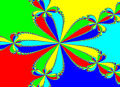# 請問 函數 圖像 對稱的一題問題

## 請問 函數 圖像 對稱的一題問題

(A) 關於x軸對稱
(B) 關於y軸對稱
(C) 關於原點對稱
(D) 關於直線 y=x 對稱

TOP

 tsusy 寸絲發短消息 加為好友 當前離線 2# 大 中 小 發表於 2012-2-24 17:37  只看該作者 令 $$b=0$$, 則 $$2f(a)=2f(a)f(0)\Rightarrow f(0)=1$$ 或 $$f(x)\equiv0$$. 若 $$f(0)=1$$, 令 $$a=0$$, 則 $$f(b)+f(-b)=2f(0)f(b)=2f(b)\Rightarrow f(b)=f(-b)$$. 所當 $$f(0)=1$$ 時，是偶函數，對稱於 y 軸。 當 $$f(0)\neq 1$$ 時，是零函數，還是偶函數，仍是對稱於 y 軸。 所以 (B) 選項正確。 例子： $$f(x)\equiv1$$ 的常數函數，此函數滿足條件，但不滿足 ACD 所以僅有 (B) 是正確的。 網頁方程式編輯 imatheq UID981 帖子1027 閱讀權限10 來自方寸之地 在線時間2944 小時 註冊時間2011-10-10 最後登錄2021-10-15  查看個人網站 查看詳細資料 TOP
﻿